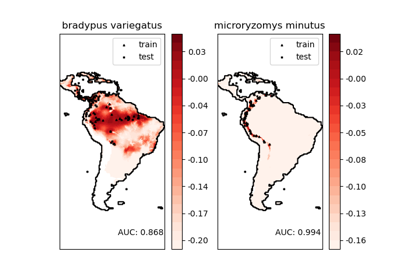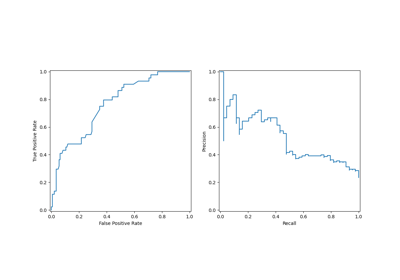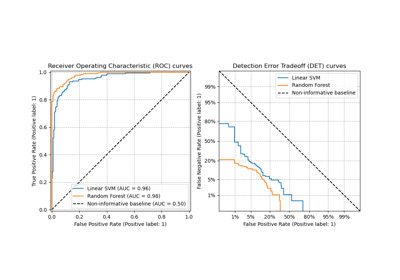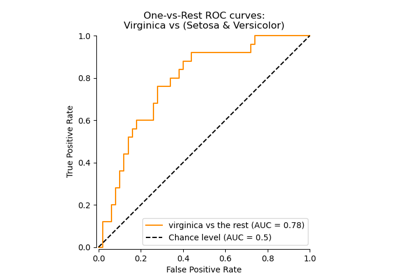# sklearn.metrics.roc_curve¶

sklearn.metrics.roc_curve(y_true, y_score, *, pos_label=None, sample_weight=None, drop_intermediate=True)[source]

Note: this implementation is restricted to the binary classification task.

Read more in the User Guide.

Parameters:
y_truearray-like of shape (n_samples,)

True binary labels. If labels are not either {-1, 1} or {0, 1}, then pos_label should be explicitly given.

y_scorearray-like of shape (n_samples,)

Target scores, can either be probability estimates of the positive class, confidence values, or non-thresholded measure of decisions (as returned by “decision_function” on some classifiers).

pos_labelint, float, bool or str, default=None

The label of the positive class. When pos_label=None, if y_true is in {-1, 1} or {0, 1}, pos_label is set to 1, otherwise an error will be raised.

sample_weightarray-like of shape (n_samples,), default=None

Sample weights.

drop_intermediatebool, default=True

Whether to drop some suboptimal thresholds which would not appear on a plotted ROC curve. This is useful in order to create lighter ROC curves.

New in version 0.17: parameter drop_intermediate.

Returns:
fprndarray of shape (>2,)

Increasing false positive rates such that element i is the false positive rate of predictions with score >= thresholds[i].

tprndarray of shape (>2,)

Increasing true positive rates such that element i is the true positive rate of predictions with score >= thresholds[i].

thresholdsndarray of shape (n_thresholds,)

Decreasing thresholds on the decision function used to compute fpr and tpr. thresholds represents no instances being predicted and is arbitrarily set to np.inf.

RocCurveDisplay.from_estimator

Plot Receiver Operating Characteristic (ROC) curve given an estimator and some data.

RocCurveDisplay.from_predictions

Plot Receiver Operating Characteristic (ROC) curve given the true and predicted values.

det_curve

Compute error rates for different probability thresholds.

roc_auc_score

Compute the area under the ROC curve.

Notes

Since the thresholds are sorted from low to high values, they are reversed upon returning them to ensure they correspond to both fpr and tpr, which are sorted in reversed order during their calculation.

An arbitrary threshold is added for the case tpr=0 and fpr=0 to ensure that the curve starts at (0, 0). This threshold corresponds to the np.inf.

References



Fawcett T. An introduction to ROC analysis[J]. Pattern Recognition Letters, 2006, 27(8):861-874.

Examples

>>> import numpy as np
>>> from sklearn import metrics
>>> y = np.array([1, 1, 2, 2])
>>> scores = np.array([0.1, 0.4, 0.35, 0.8])
>>> fpr, tpr, thresholds = metrics.roc_curve(y, scores, pos_label=2)
>>> fpr
array([0. , 0. , 0.5, 0.5, 1. ])
>>> tpr
array([0. , 0.5, 0.5, 1. , 1. ])
>>> thresholds
array([ inf, 0.8 , 0.4 , 0.35, 0.1 ])


## Examples using sklearn.metrics.roc_curve¶Species distribution modeling

Species distribution modelingVisualizations with Display Objects

Visualizations with Display Objects